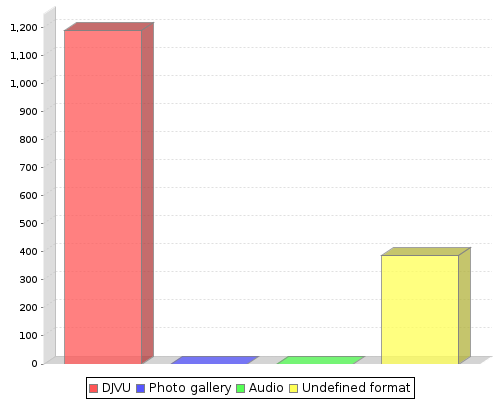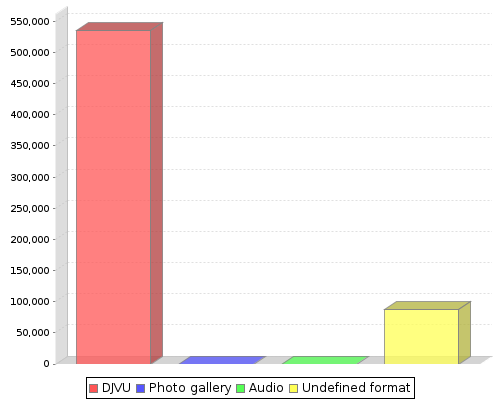Number of objects: 355

Total number of users since Oct 26, 2020: 11428

Below there are statistics of objects attribute values. Statistics are gathered since 2020-10-27 19:43:13

Number of new objects added in different formatsThe number in parentheses is the total number of objects with content for a given format

1. PDF 
2. Undefined format 
Number of content views for different formatsThe number in parentheses is the total number of content views requested for a given format

1. PDF 
2. Undefined format 

Below there are statistics of objects attribute values. Statistics are gathered since Oct 26, 2020

Choose attribute:

Ranking by total number of objectsNumber in brackets is a number of all objects which metadata description contains given attribute value.

1. Article 
2. Commentary 
3. Introduction 
4. Contents 
Ranking by number of viewed objectsNumber in brackets is a sum of all reads of objects which metadata description contains given attribute value.

1. Commentary 
2. Contents 
3. Article 
4. Introduction